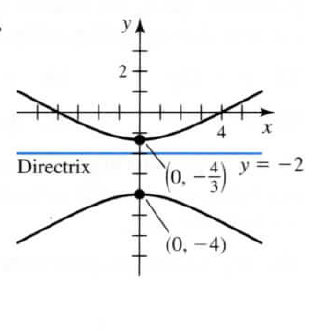×
Get Full Access to Calculus: Early Transcendentals - 1 Edition - Chapter 10.4 - Problem 73e
Get Full Access to Calculus: Early Transcendentals - 1 Edition - Chapter 10.4 - Problem 73e

×

# Answer: Graphs to polar equations Find a polar equationISBN: 9780321570567 2

## Solution for problem 73E Chapter 10.4

Calculus: Early Transcendentals | 1st Edition

• Textbook Solutions
• 2901 Step-by-step solutions solved by professors and subject experts
• Get 24/7 help from StudySoup virtual teaching assistantsCalculus: Early Transcendentals | 1st Edition

4 5 1 309 Reviews
22
2
Problem 73E

Find a polar equation for each conic section.Step-by-Step Solution:

Solution 73EStep 1:Graphs to polar equations Find a polar equation for each conic section. The polar equation of the hyperbola is of the form Where is the eccentricity and is the directrix.In the given graph,it is given that the directrix Therefore Since it is an hyperbola then we have Therefore the polar equation of the given diagram is Step 3:Now we have to find the eccentricity From the figure we can find that And Thus on solving this we get and Now we know that the expression for directrix of an ellipse is It is given in the picture that the directrix is Therefore Step 4:Therefore the polar equation of the given diagram is

Step 2 of 4

Step 3 of 4

##### ISBN: 9780321570567

Unlock Textbook Solution